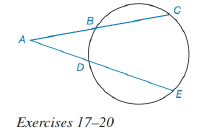Chapter 6.3, Problem 20E### Elementary Geometry for College St...

6th Edition
Daniel C. Alexander + 1 other
ISBN: 9781285195698

#### Solutions

Chapter
Section### Elementary Geometry for College St...

6th Edition
Daniel C. Alexander + 1 other
ISBN: 9781285195698
Textbook Problem
1 views

# For Exercises 17 to 2, See Theorem 6.3.6.Given: A B = 5 , B C = 6 , A D = 6 Find: AE.To determine

To find:

The value of AE

Explanation

The diagrammatic representation is given below,

Theorem:

If secant segments are drawn to a circle from an external point, then the products of the length of each secant with the length of its external segment are equal.

By using the theorem 6.3.5 to get the following,

We know that

AC=AB+BCAC=5+6AC=11

### Still sussing out bartleby?

Check out a sample textbook solution.

See a sample solution

#### The Solution to Your Study Problems

Bartleby provides explanations to thousands of textbook problems written by our experts, many with advanced degrees!

Get Started

#### Find more solutions based on key concepts